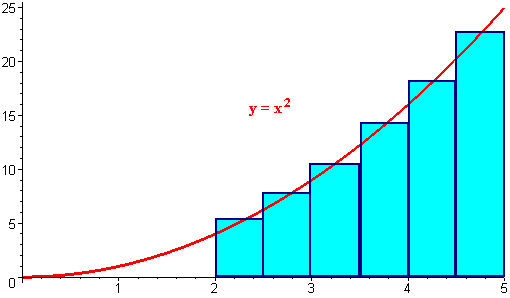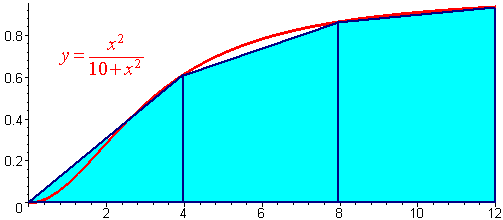$$\newcommand{\id}{\mathrm{id}}$$ $$\newcommand{\Span}{\mathrm{span}}$$ $$\newcommand{\kernel}{\mathrm{null}\,}$$ $$\newcommand{\range}{\mathrm{range}\,}$$ $$\newcommand{\RealPart}{\mathrm{Re}}$$ $$\newcommand{\ImaginaryPart}{\mathrm{Im}}$$ $$\newcommand{\Argument}{\mathrm{Arg}}$$ $$\newcommand{\norm}{\| #1 \|}$$ $$\newcommand{\inner}{\langle #1, #2 \rangle}$$ $$\newcommand{\Span}{\mathrm{span}}$$

# Numerical Integration

$$\newcommand{\vecs}{\overset { \rightharpoonup} {\mathbf{#1}} }$$ $$\newcommand{\vecd}{\overset{-\!-\!\rightharpoonup}{\vphantom{a}\smash {#1}}}$$$$\newcommand{\id}{\mathrm{id}}$$ $$\newcommand{\Span}{\mathrm{span}}$$ $$\newcommand{\kernel}{\mathrm{null}\,}$$ $$\newcommand{\range}{\mathrm{range}\,}$$ $$\newcommand{\RealPart}{\mathrm{Re}}$$ $$\newcommand{\ImaginaryPart}{\mathrm{Im}}$$ $$\newcommand{\Argument}{\mathrm{Arg}}$$ $$\newcommand{\norm}{\| #1 \|}$$ $$\newcommand{\inner}{\langle #1, #2 \rangle}$$ $$\newcommand{\Span}{\mathrm{span}}$$ $$\newcommand{\id}{\mathrm{id}}$$ $$\newcommand{\Span}{\mathrm{span}}$$ $$\newcommand{\kernel}{\mathrm{null}\,}$$ $$\newcommand{\range}{\mathrm{range}\,}$$ $$\newcommand{\RealPart}{\mathrm{Re}}$$ $$\newcommand{\ImaginaryPart}{\mathrm{Im}}$$ $$\newcommand{\Argument}{\mathrm{Arg}}$$ $$\newcommand{\norm}{\| #1 \|}$$ $$\newcommand{\inner}{\langle #1, #2 \rangle}$$ $$\newcommand{\Span}{\mathrm{span}}$$

Review of Left and Right Sums

## The Midpoint Approximation

To get a better approximation of , for example,

$\int_2^5x^2 \, dx$

we could instead use the y-value of the midpoint of each interval as the height. We will use six rectangles.Notice that the first midpoint is at 2.25 and each rectangle width

$\Delta x = \dfrac{5-2}{6} = 0.5.$

The new numbers are as follows:

$2.25 + 0(0.5), 2.25 + 1(0.5), 2.25 + 2(0.5), 2.25 + 3(0.5), 2.25 + 4(0.5), 2.25 + 5(0.5)$

so that the y-coordinates are

$(2.25 + 0(0.5))^2, (2.25 + 1(0.5))^2, (2.25 + 2(0.5))^2, (2.25 + 3(0.5))^2, (2.25 + 4(0.5))^2, (2.25 + 5(0.5))^2.$

We see that the ith rectangle has y-coordinate:

$$\text{height} = (2.25 + i(.5))^2$$.

To get the area of the ith rectangle we multiply the height by the base:

$$(2.25 + i(.5))^2(.5)$$.

Finally to get the total area we add the terms up:

$$S(2.25 + i(.5))^2(.5) = 38.9975$$.

The true solution is 37.66... . The left endpoint approximation would have yielded 33.875 and the right endpoint approximation would have yielded 44.375. We see that for this case, the midpoint approximation yields a closer approximation.

This approximation is called the midpoint approximation and is given in general by:

Definition: The Midpoint Approximation

$\int_{a}^{b} f(x) dx \approx \dfrac{b-a}{n} \sum_{i=1}^n f(\dfrac{x_{i-1}+x_i}{2}) = \dfrac{b-a}{n}\sum_{i=1}^n f\left(\dfrac{b-a}{2n}+\dfrac{b-a}{n}i \right).$

## The Trapezoidal Approximation

A fourth method involves the trapezoidal rule which geometrically calculates the area of the trapezoid with base on the x-axis and heights $$f(x_i)$$ and f(x_{i+1}). The area of the trapezoid is

$\dfrac{\Delta x}{2} \left[ f(x_i) + f(x_{i+1}) \right]$

or the base times the average of the heights. Adding up all the trapeziods gives:

Definition: The Trapezoidal Approximation

$T(n) = \dfrac{b-a}{2n} \left[f(x0) + 2f(x_1) + 2f(x_2) +...+ 2f(x_{n-1}) + f(x_n) \right].$

Example $$\PageIndex{1}$$: Trapezoidal Approximation

Use the trapezoidal approximation with three trapezoids to approximate the integral

$\int_0^{12} \dfrac{x^2}{10+x^2} dx.$

Solution

The picture is shown below.The x values of interest are

$$x_0 = 0$$, $$x_1 = 4$$, $$x_2 = 8$$, $$x_3 = 12$$.

Plugging in these values into the function gives

$$f(0) = 0$$, $$f(4) = 0.6154$$, $$f(8) = 0.8649$$, $$f(12) = 0.9351$$.

The trapezoid approximation formula gives

$T(n) = \dfrac{12 - 0}{2} \left [0 + 2(0.6154) + 2(0.8649) + 0.9351 \right]$

$= 7.7914.$

We can compare this with the true answer of 7.8475.

Exercise $$\PageIndex{1}$$:

Use the Trapezoidal Approximation with 5 trap to approximate the integral

$\int_3^13 \sqrt{1+x^3}\, dx.$

## Error

The error in approximating an integral can be found by subtracting the true value from the estimated value. The graphs show that the error is directly inked to the concavity of the integrand. Without proof, bounds for the errors using the midpoint and trapezoid approximations are:

$|E_M| \le \dfrac{B(b - a)^3 }{24n^2}$

$|E_T| \le \dfrac{B(b - a)^3 }{12n^2}.$

Where

$B = \text{max} |f ''(x)|.$

Example $$\PageIndex{2}$$

If you want to approximate

$\int _0^2 e^{x^2} \, dx$

using the midpoint rule with an error of less than 0.001, we compute

$f''(x) = \left(4+4x^2\right)e^{x^2}$

which in an increasing function on $$[0,2]$$, hence has its maximum at $$x = 2$$. So

$B = (2 + 4(4))e^4 < 983$

so we find

$\dfrac{983(2^3)}{24n^2} < 0.001$

or

$7864 < 0.001(24n^2)$

$n^2 < 327667.$

Taking square roots of both sides gives

$n > 572.$

Hence if we let

$n = 573$

we are guaranteed to have an error less than 0.001.

## Simpson's Estimate

We saw that the Trapezoidal and Midpoint estimates provided better accuracy than the Left and Right endpoint estimates. It turns out that a certain combination of the Trapezoid and Midpoint estimates is even better.

Definition: Simpson's Approximation

Let $$f(x)$$ be a function defined on $$[a,b]$$. Then

$S(n) = \dfrac{T(n)}{3} + \dfrac{2M(n)}{3}$

where $$T(n)$$ and $$M(n)$$ are the Trapezoidal and Midpoint Estimates. $$S(n)$$ is called Simpson's Estimate for the integral.

Geometrically, if $$n$$ is an even number then Simpson's Estimate gives the area under the parabolas defined by connecting three adjacent points. Let $$n$$ be even then using the even subscripted $$x$$ values for the trapezoidal estimate and the midpoint estimate, gives

\begin{align} S(n) &= \dfrac{1}{3}\Big[\dfrac{(b-a)}{2n} \big( f(x_0) +2f(x_2) +...+ f(x_{2n-2}) +f(x_{2n}) \big)\Big] \\ &\;\;\; + \dfrac{2}{3}\Big[\dfrac{b-a}{n}\big( f(x_1)f(x_3)+f(x_5)+...+f(x_{2n-1}) \big) \Big] \\ &= \dfrac{b-a}{3n}\Big(f(x_0)+4f(x_1)+2f(x_2)+4f(x_3)+2f(x_4)+4f(x_5)+...+2f(x_{n-2})+4f(x_{n-1})+f(x_n) \Big) \end{align}

Notice the
1 2 4 2 4 ... 2 4 2 4 1
pattern.

Example $$\PageIndex{3}$$: Simpson's Approximation

Use Simpson's Approximation with $$n = 6$$ to approximate $\int_1^4 \dfrac{1}{1+x^3} dx$

Solution

The key values of $$x$$ are

$$x_0 = 1$$, $$x_1 = 1.5$$, $$x_2 = 2$$, $$x_3 = 2.5$$, $$x_4 = 3$$, $$x_5 = 3.5$$, $$x_6 = 4$$

and the function values are

$$f(x_0) = 0.5$$, $$f(x_1) = 0.2286$$, $$f(x_2) = 0.1111$$,

$$f(x_3) = 0.0602$$, $$f(x_4) = 0.0357$$, $$f(x_5) = 0.0228$$, $$f(x_6) = 0.0154$$.

Now we can put these numbers into the Simpson's approximation formula.

$S(n) = \dfrac{4 - 1}{3(6)} \left(0.5 + 4(0.2286) + 2(0.1111) + 4(0.0602) + 2(0.0357) + 4(0.0228) + 0.0154 \right)$

$= 0.3426.$

Exercise $$\PageIndex{3}$$: Simpson's Approximation

Use Simpson's approximation with $$n = 4$$ to approximate

$\int _2^18 \dfrac{1}{x}\,dx.$

## Error in Simpson's Estimate

Without proof, we state

Let

$M = \text{max} |f''''(x)|$

and let $$E_S$$ be the error in using Simpson's estimate. Then

$|E_S| \le \dfrac{M(b - a)^5 }{180n^4}.$

Example $$\PageIndex{4}$$:

Determine the value of n that will approximate

$\int _1^3 \dfrac{1}{x} dx$

within two decimal places of accuracy.

Solution

We need to have an error less than 0.005:

$|E_S| < 0.005.$

We have

$b - a = 3 - 1 = 2.$

We take derivatives to compute $$M$$:

$f(x) = \dfrac{1}{x}$

$f '(x) = \dfrac{-1}{x^2}$

$f ''(x) = \dfrac{2}{x^3}$

$f'''(x) = \dfrac{-6}{x^4}$

$f''''(x) = \dfrac{24}{x^5}.$

We see that the maximum value of this function between 1 and 3 occurs when $$x = 1$$:

$M = |24/1^5| = 24 .$

We put this together to get

$\dfrac{(24)(2^5)}{180n^4} < 0.005.$

Multiplying by the denominator gives

$768 < 0.9 n^4$

or

$853.33 < n^4.$

Taking fourth roots gives

$n > 5.4.$

Hence, if we choose $$n = 6$$ we are guaranteed two decimals of accuracy.

Exercise $$\PageIndex{4}$$:

If you want to approximate

$\int _0 ^1 \cos \,x \, dx$

with $$n = 5$$, determine the maximum errors that occur using the midpoint, the trapezoidal, and Simpson's approximation.

Larry Green (Lake Tahoe Community College)